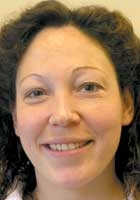27 March 2007

Working at the interface between physics and mathematics

What are the current areas of research you work in?

Nina studied theoretical physics as an undergraduate in Canada and was fascinated by quantum mechanics. “Quantum mechanics is a beautiful theory and so different from intuitive classical mechanics,” she says. “I was interested in chaotic systems so I chose to come to Bristol to do my PhD as it has an excellent reputation for research in quantum chaos.” How large objects, such as balls on a billiard table, interact in a dynamic system can be relatively easily modelled using Newton’s classic laws of motion. “In a classical chaotic system, however, it is like modelling balls on a billiard table that no longer has a straight edge but is irregular. This makes prediction of speed or direction of the balls very complicated,” explains Nina. “If you imagine the table getting smaller and smaller, reducing to the size of atoms, then the laws of classical mechanics no longer apply and quantum mechanics takes over.” At the quantum level Heisenberg’s uncertainty principle means that you cannot determine both the position and velocity of a particle, and at this small scale the energy levels of particles, such as electrons, are quantized. This means that only certain discrete energies are allowed. The field of quantum chaos involves understanding how these quantum objects behave in systems with chaotic classical analogues, and determining methods that give information such as the distribution of these quantum energies.

How do you work?

Nina has a traditional approach to mathematics. “I am very much a pencil and paper mathematician,” she admits. “My research involves a lot of working with, and thinking about, formulae, but there is also a lot of interaction with other people – exchanging ideas and getting different views.” The main part of Nina’s work is on Random Matrix Theory – a technique used to model quantum chaos, which is now being applied to fundamental research in number theory. “Usually maths supports physics but this is an example where a technique developed in physics is pushing things forward in maths,” Nina explains. She is applying Random Matrix Theory to the Riemann zeta function, an object of fundamental importance in number theory. In fact, solving the Riemann hypothesis is so important it has been designated one of the ‘Millennium prize problems’. These are seven mathematical problems that have so far resisted solution, thus a million-dollar prize is attached to solving each one. “Number theory is the theory of arithmetic, literally the theory of whole numbers or integers – understanding how prime numbers arise and other fundamental concepts. The Riemann zeta function, as with other functions, can be thought of as describing a land-scape. I am investigating the contours of this landscape for unusual features: in particular the places where the  function is zero. The link with Random Matrix Theory is that this also has features that can be interpreted as zeros and by comparing the distribution of zeros in the two systems new insights can be obtained, new questions asked and our understanding of both systems improved.”

The connection between number theory and random matrix theory was made during a casual conversation over tea between a physicist and a mathematician in the 1970s and one of the main benefits of Nina’s fellowship has been the ability to travel and discuss results and techniques with number theorists. “Bristol is full of experts on quantum chaos but, until the last couple of years, there weren’t many number theorists,” says Nina. “Now the mathematics department has a very strong number theory group, but at a time when there was less local expertise I was able to spend 15 months in the US at a major math-ematics institute working with number theorists, essentially understanding the right questions to ask. It is very much a two-way street for information between number theory and random matrix theory, which makes the interaction even more exciting.”

How will your work help society?

Number theory is a vital area for security in IT, ensuring, for example, that internet transactions are secure. Random Matrix Theory is a rapidly growing field in physics and mathe-matics. It is used in nuclear and solid state physics. “Interestingly, it is now becoming possible, thanks to nano-technology, to fabricate systems that effectively create quantum billiard tables,” says Nina. These nanosystems will be able to test the effects predicted by Random Matrix Theory and should lead to new insights, improved theory and therefore to the design and production of new materials with unusual electronic and other properties.

Do you think studying postgraduate mathematics is becoming more popular?

Nina sees her area of work as being a very cool area of research. “There is certainly no shortage of graduates wanting to study at Bristol,” she concludes. “Random Matrix Theory and Number Theory are extremely hot topics, frontier work in fact, and we get some exceptional students inspired to get involved with the work.”

Dr Nina Snaith’s Fellowship is funded by the Engineering and Physical Sciences Research Council.